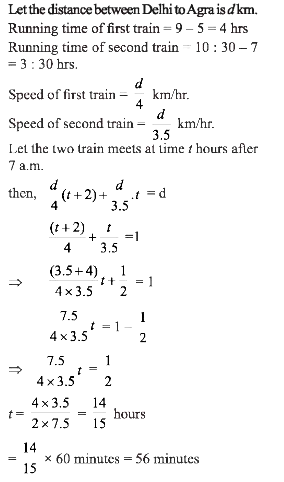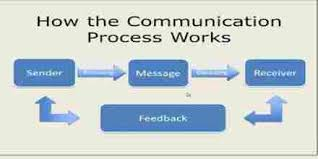# UGC NET PAPER 1 June Shift (Pack of 3) - UGC NET PAPER 1 26th June 2019 Morning Shift

>>>>>>>>UGC NET PAPER 1 26th June 2019 Morning Shift

• A

6:36 a.m.• B

6:56 a.m.• C

7 a.m.• D

756 a.m.• Option : D
• Explanation :• A

158• B

265• C

367• D

375• Option : B
• Explanation : Let the number is n, then its reciprocal is 1/n.
Now, the sum of the numbers = n + 1/n
= (n2 + 1)/n
Now, from the options (a), when denominator
n = 8
then, numerator should be
(n)2 + 1 = (8)2 + 1 = 65
Option (b) when denominator n = 5
then, numerator should be n2 + 1 = 52 + 1 = 26.
Hence, fraction is 26/5.

• A

The sender transmits the message• B

The message enters the channel• C

The message leaves the channel• D

The receiver understands the message• Option : D
• Explanation : As the figure below depicts, the communication process is complete only when the receiver decodes or understands the sent message.• A

(i) only• B

(ii) only• C

(i), (ii) and (iii)• D

(ii) and (iii)• Option : C
• Explanation : The given proposition are 'All republics are grateful'
It's negative proposition are 'No republics are grateful'
or 'Republics are not grateful' . This is equivalent to 'Some republics are not grateful'.

• A

Hypothetical• B

Deductive• C

Inductive• D

Analogical• Option : D
• Explanation : Analogical reasoning relies upon analogy, citing accepted similarities between two systems to support the conclusion that some further similarity exists. In the given argument, the term 'jus as' clearly shows the comparison between the pre-active stage of classroom teaching and pre-learning preparation stage of communication.